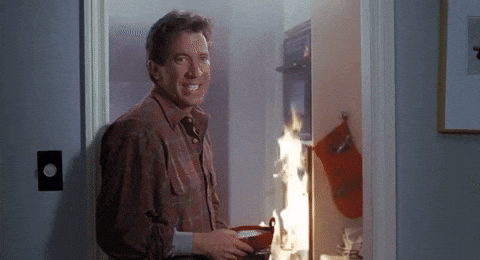# Thanksgiving Gantt Chart

Thanksgiving 🦃 is right around the corner 🎉 – this year we are hosting 17 people 😱. If you too are hosting way more than your kitchen normally cooks for, perhaps this will be of interest!
rstats
thankyou
Author

Lucy D’Agostino McGowan

Published

November 12, 2017

Thanksgiving 🦃 is right around the corner 🎉 – this year we are hosting 17 people 😱.

Ingredients:
📦 lubridate
📦 plotly

If you too are hosting way more than your kitchen normally cooks for, perhaps this will be of interest! We decided to make a Google Sheet of the various dishes so we could plot out what will be cooking when.

## Packages we’ll use

``````library(googlesheets4)
library(lubridate)
library(plotly)``````

## Pull in the data

First we can pull the spreadsheet into R using the googlesheets package.

``````## Read the sheet into R

## Clean up a bit 🛀

``````dishes_df\$start <- as_datetime(dishes_df\$start,
tz = "America/Chicago")
dishes_df\$finish <- as_datetime(dishes_df\$finish,
tz = "America/Chicago")
dishes_df\$minutes <- dishes_df\$finish - dishes_df\$start``````

Let’s pick some lovely turkey-themed colors for our chart 🌈 brought to you by colour lovers.

``````cols <- c("#487878", "#783030", "#904830", "#A87860", "#D89048")
dishes_df\$color  <- factor(dishes_df\$where, labels = cols)

## Order for the chart
dishes_df <- dishes_df[order(dishes_df\$start,
decreasing = TRUE), ]``````

## Make the plot 💃

This is inspired by a Plotly blog post!

``````p <- plot_ly()

for (i in 1:nrow(dishes_df)) {
x = c(dishes_df\$start[i], dishes_df\$finish[i]),
y = c(i, i),
line = list(color = dishes_df\$color[i],
width = 20),
hoverinfo = "text",
text = paste("Dish: ",
dishes_df\$dish[i],
"<br>",
"Cook time: ",
dishes_df\$minutes[i],
"minutes<br>",
"Where: ",
dishes_df\$where[i]),
showlegend = FALSE
)
}````````````## Add the dish names to the y-axis, remove grid
p <- layout(p,
xaxis = list(showgrid = FALSE),
yaxis = list(showgrid = FALSE,
tickmode = "array",
tickvals = 1:nrow(dishes_df),
ticktext = unique(dishes_df\$dish)),
margin = list(l = 200, r = 50, b = 50, t = 50, pad = 4),
plot_bgcolor = "#EBE5E5",
paper_bgcolor = "#EBE5E5",
## add a turkey because why not!
images = list(